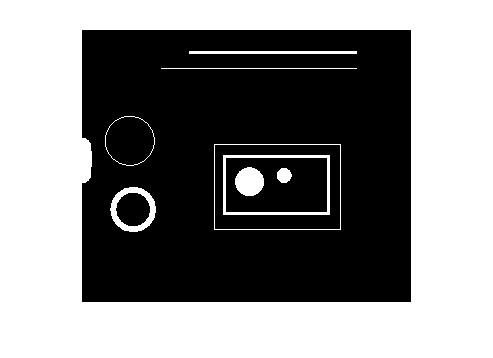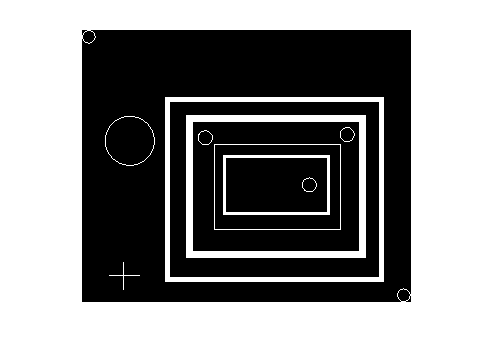# Using ismember with the output of regionprops

I recently heard a MathWorks application engineer (hi, Brett!) say that a short little paragraph in the regionprops documentation was very "powerful." Here it is:

The function ismember is useful in conjunction with regionprops for selecting regions based on certain criteria. For example, these commands create a binary image containing only the regions whose area is greater than 80.

   idx = find([stats.Area] > 80);
BW2 = ismember(L,idx);

When I heard Brett's comment, I immediately pulled out my high-tech note-taking device (index card) from my back pocket and wrote: "regionprops - ismember - blog topic."

The basic idea is this: If I have a binary image containing objects, how can I make a new binary image containing a subset of the objects satisfying certain criteria? The answer is in three parts:

1. Use bwlabel and regionprops to compute measurements of the objects.

2. Compute a vector of labels for the objects satisfying the criteria. Sometimes a special structure syntax called the "comma-separated list" is very useful for this step; I'll show that syntax below.

3. Use ismember to compute the desired binary image.

I've posted several examples using bwlabel and regionprops, so I won't go into much detail about them. You label objects in a binary image using bwlabel, and then you compute geometric measurements of the labeled objects using regionprops.

Let's use the blobs.png image for our example.

bw = imread('blobs.png');
imshow(bw)L = bwlabel(bw);
s = regionprops(L, 'Area', 'BoundingBox');
s(1)
ans =

Area: 35
BoundingBox: [0.5000 0.5000 14 14]



regionprops returns a structure array containing the desired measurements for each object.

The comma-separated list syntax for structures offers an easy way to convert a particular field from a structure array into a vector, assuming that the field value is always a scalar. Here's how it works. When s is a structure array with field foo, and you write the following expression:

   s.foo

MATLAB translates that into this "comma-separated list":

   s(1).foo, s(2).foo, ..., s(end).foo

Therefore, if s(k).foo is a scalar, you can create a vector of all the foo values by enclosing s.foo in brackets, like this:

   foo_fector = [s.foo];

Here's how it works for the 'Area' measurements we computed above for the blobs.png image.

area_values = [s.Area]
area_values =

Columns 1 through 7

35         393          19         139          36          58         736

Columns 8 through 14

53           2           2           1           2           2           2

Columns 15 through 21

1         196        3940        4326         504          40         422

Columns 22 through 28

966         655         175          40          39        1108          35



Suppose we want a list of all the objects whos area is between 100 and 1000. We can do that using find and relational operators, like this:

idx = find((100 <= area_values) & (area_values <= 1000))
idx =

2     4     7    16    19    21    22    23    24



And that brings us to ismember. We can construct a binary image containing all the objects whos area is between 100 and 1000 by passing L and idx to ismember.

bw2 = ismember(L, idx);
imshow(bw2)Let's try that with another measurement, solidity. Solidity is defined as the area of the region divided by the area of the convex hull of the region.

s = regionprops(L, {'Solidity'});
solidity = [s.Solidity]
solidity =

Columns 1 through 9

0.2397    0.9680    0.6786    0.0700    0.8000    0.1321    0.4461    0.7910    1.0000

Columns 10 through 18

1.0000    1.0000    1.0000    1.0000    1.0000    1.0000    1.0000    0.0972    0.1681

Columns 19 through 27

1.0000    0.2235    0.0386    0.1505    0.9791    0.9669    0.2260    0.2191    0.5604

Column 28

0.2397



Here are all the objects with a solidity of 0.9 or higher:

imshow(ismember(L, find(solidity >= 0.9)))And here are all the objects with a solidity of 0.25 or lower:

imshow(ismember(L, find(solidity <= 0.25)))Published with MATLAB® 7.7

|